# Texas Go Math Grade 7 Lesson 2.2 Answer Key Constant Rates of Change

Refer to our Texas Go Math Grade 7 Answer Key Pdf to score good marks in the exams. Test yourself by practicing the problems from Texas Go Math Grade 7 Lesson 2.2 Answer Key Constant Rates of Change.

## Texas Go Math Grade 7 Lesson 2.2 Answer Key Constant Rates of Change

A giant tortoise moves at a slow but steady pace. It takes the giant tortoise 3 seconds to travel 10.5 inches.A. Use the bar diagram to help you determine how many inches a tortoise travels in 1 second. What operation did you use to find the answer?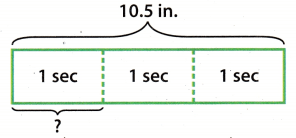B. Complete the table.C. For each column of the table, find the distance and the time. Write each fraction as a decimal. Put distance in the numerator and time in the denominator.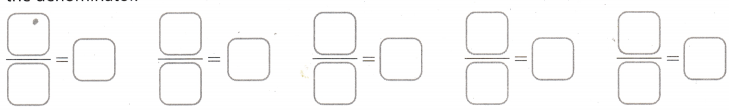D. What do you notice about the decimal forms of the fractions?

E. Conjecture How do you think the distance a tortoise travels is related to the time?

Reflect

Question 1.
Suppose the tortoise travels for 12 seconds. Explain how you could find the distance the tortoise travels.
We could simply multiply tortoises unit rate of inches per second with 12.

Question 2.
How would you describe the rate of speed at which a tortoise travels?
We would describe it with a rate of inches per second.

Question 3.
The table shows the distance Allison drove on one day of her vacation. Is the relationship between the distance and the time a proportional relationship? Did she drive at a constant speed? Explain.Write the rates.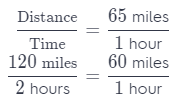We can stop here, and see that the rates are different. Thus, she did not drive at a constant speed.

Question 4.
For a school field trip, there must be 1 adult to accompany 12 students, 3 adults to accompany 36 students, and 5 adults to accompany 60 students. Show that the relationship between the number of adults and the number of students is a proportional relationship. Then write an equation for the relationship.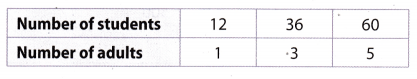Write the rates.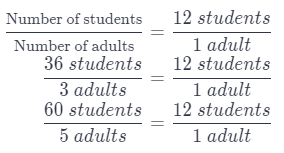The rates are equaL They are constant so the relationship is proportional
Let x represent the number of students.
Let y represent the number of adults.
The equation for the relationship is:
y = $$\frac{x}{12}$$

Question 1.
Based on the information in the table, is the relationship between time and the number of words typed a proportional relationship? (Explore Activity and Example 1)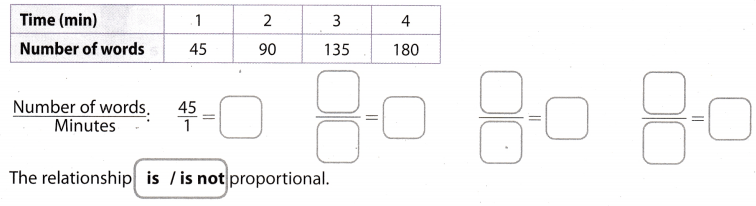The relationship is proportional.

Find the constant of proportionality k. Then write an equation for the relationship between x and y. (Example 2)

Question 2.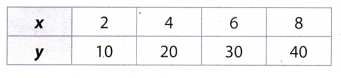$$\frac{y}{x}$$ : = $$\frac{10}{2}$$ = 5 ; $$\frac{30}{6}$$ = 5 ; $$\frac{40}{8}$$ = 5
k = 5
Equation: y = 5x
k = 5, y = 5x

Question 3.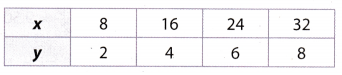$$\frac{x}{y}$$ : = $$\frac{8}{2}$$ = 4 ; $$\frac{16}{4}$$ = 4 ; $$\frac{24}{6}$$ = 4 ; $$\frac{32}{8}$$ = 4
k = 4
Equation: x = 4y
k = 4, x = 4y

Essential Question Check-In

Question 4.
How can you represent a proportional relationship using an equation?
We represent the proportional relationship using a constant that is a ratio of two variables.

Information on three car-rental companies is given.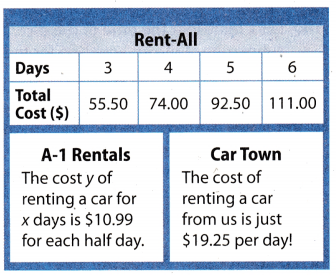Question 5.
Write an equation that gives the cost y of renting a car for x days from Rent-All. _______________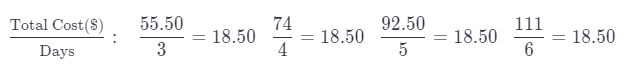y = 18.5x

Question 6.
What is the cost per day of renting a car from A-1? ___________
If cost for half a day is equal to $10.99, then cost per day is 2 times greater:$10.99 × 2 = $21.98 per day Question 7. Analyze Relationships Which company offers the best deal? Why? Answer: Rent-all offers the best deal, because it’s price of car rental per day is the lowest. Question 8. Critique Reasoning A skydiver jumps out of an airplane. After 0.8 second, she has fallen 100 feet. After 3.1 seconds, she has fallen 500 feet. Emtiaz says that the skydiver should fall about 187.5 feet in 1.5 seconds. Is his answer reasonable? Explain. Answer: $$\frac{\text { Distance(feet) }}{\text { Time(seconds) }}: \frac{100}{0.8}=125 \quad \frac{500}{3.1}=161.3 \quad \frac{187.5}{1.5}=125$$ Emtiazs answer is not reasonable because longer the skydiver falls, faster he should be. So, he should have taken in consideration a constant somewhere between first and second, not the first. Steven earns extra money babysitting. He charges$31.25 for 5 hours and $50 for 8 hours. Question 9. Explain why the relationship between how much Steven charges and time is a proportional relationship. Answer: $$\frac{\text { Price }(\)}{\text { Time(hours) }}: \quad \frac{31.25}{5}=6.25 \quad \frac{50}{8}=6.25$$ The relationship is proportional, because the price per hour in both case is equal. Question 10. Interpret the Answer Explain what the constant rate of change means in the context. Answer: The constant rate of change represents price per hour. Question 11. Write an equation to represent the relationship. Tell what the variables represent. Answer: x = Time (hours) y = Price ($)
y = 6.25x

Question 12.
How much would Steven charge for 3 hours?
Multiply unit rate of price per hour by 3:
3 × 6.25 = $18.75 Steven would charge$18.75 for 3 hours.

A submarine dives 300 feet every 2 minutes, and 6,750 feet every 45 minutes.

Question 13.
Find the constant rate at which the submarine dives, Give your answer in feet per minute and in feet per hour.Submarine dives 150 feet per minute or 9000 feet per hour.

Question 14.
Let x represent the time of the dive. Let y represent the depth of the submarine. Write an equation for the proportional relationship using the rate in feet per minute.
y = 150x

Question 15.
Draw Conclusions If you wanted to find the depth of a submarine during a dive, would it be more reasonable to use an equation with the rate in feet per minute or feet per hour? Explain your reasoning.
It all depends what is more comfortable to use.
E.g.
If we want to know where the submarine is after 3 hours, we would use feet per hour.
If we want to know where the submarine is after 38 minutes, we would use feet per minute.

H.O.T. Focus On Higher Order Thinking

Question 16.
Make a Conjecture There is a proportional relationship between your distance from a thunderstorm and the time from when you see lightning to when you hear thunder. If there are 9 seconds between lightning and thunder, the storm is about 3 kilometers away. If you double the amount of time between lightning and thunder, do you think the distance in kilometers also doubles? Justify your reasoning.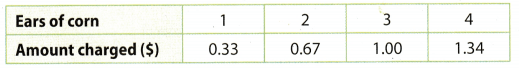$$\frac{0.33}{1}$$ = 0.33 $$\frac{0.67}{2}$$ = 0.335